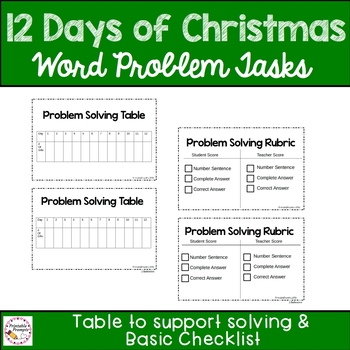DID YOU KNOW:
Seamlessly assign resources as digital activities

Learn how in 5 minutes with a tutorial resource. Try it Now

Learn More# 12 Days of Christmas Math Multiplication Problems3rd - 4th
Subjects
Standards
Resource Type
Formats Included
• PDF
Pages
38 pages

#### Also included in

1. Keep students engaged those last few days before the holidays in meaningful math instruction using the 12 days of Christmas song. Students will use a printable or digital format ( created with Google Slides) to solve multiplication and repeated addition problems in order to reveal patterns in number
\$5.00
\$6.00
Save \$1.00

### Description

Looking for a way to engage students in multiplication and word problem practice this December? This low prep resource is ready to print and is easy to use with students during math centers, or as a problem solving project this December. Reinforce multiplication as repeated addition, as well as discover the commutative property of multiplication.

Check out the printable & digital bundle which includes this resource in a time and money saving bundle!

INCLUDES:

*Teaching Notes & Suggested Uses as well as suggestions for differentiation

*Suggested lesson development

*Printable student booklet with:

-Cover page

-Words that go with the song

-Pre solving discussion questions

- Introducing the task and planning questions

-Work it out (2 student work pages)

- Table for students to complete (sample completed table at the end of the resource.

-Table for totaling up the number of each item using multiplication

*Word problems on task cards OR full page word problem pages can also be included in the resource.

*Basic problem solving checklist

*Basic table to be used for differentiation.

This would be great to use as a partner activity, performance task, or even as a math center activity!

Sure to keep your students learning right through the holidays!

This pack is ideal for 3rd graders, but some problems may be useful for 2nd or 4th grade classes as well.

Total Pages
38 pages
N/A
Teaching Duration
N/A
Report this Resource to TpT
Reported resources will be reviewed by our team. Report this resource to let us know if this resource violates TpT’s content guidelines.

### Standards

to see state-specific standards (only available in the US).
Solve multistep word problems posed with whole numbers and having whole-number answers using the four operations, including problems in which remainders must be interpreted. Represent these problems using equations with a letter standing for the unknown quantity. Assess the reasonableness of answers using mental computation and estimation strategies including rounding.
Interpret a multiplication equation as a comparison, e.g., interpret 35 = 5 × 7 as a statement that 35 is 5 times as many as 7 and 7 times as many as 5. Represent verbal statements of multiplicative comparisons as multiplication equations.
Solve two-step word problems using the four operations. Represent these problems using equations with a letter standing for the unknown quantity. Assess the reasonableness of answers using mental computation and estimation strategies including rounding.
Use multiplication and division within 100 to solve word problems in situations involving equal groups, arrays, and measurement quantities, e.g., by using drawings and equations with a symbol for the unknown number to represent the problem.
Interpret products of whole numbers, e.g., interpret 5 × 7 as the total number of objects in 5 groups of 7 objects each. For example, describe a context in which a total number of objects can be expressed as 5 × 7.